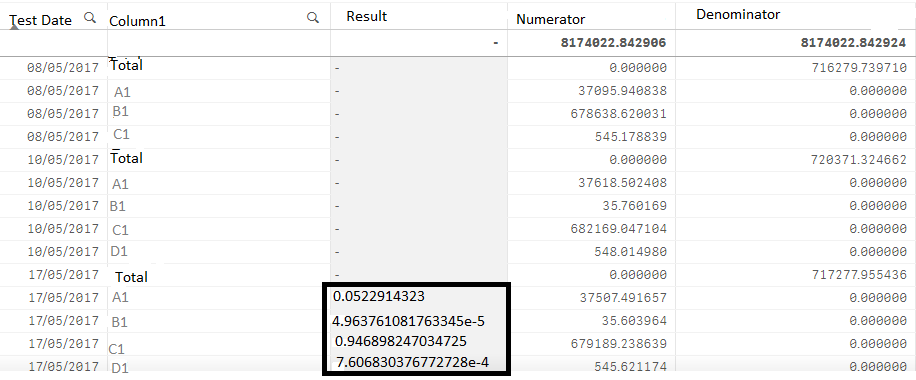QlikView App Development

Discussion Board for collaboration related to QlikView App Development.

Highlighted
New Contributor

Aggregate not working properly

Hi,

I am trying to calculate Result for the last 6 months data based on Test Date. Test Date is filter in my report.

Result for any date = Numerator for that date (A1) / Denominator for that date (Total)

So if I select Test Date as 17/05/2017, values are populating only for the selected date in filter. Other values as you see are blank in Result column. How to calculate the Result for all the dates starting from the test date selected in filter till 6 months back.

Result is getting populated only for date selected. Do we need to use Aggr like in below expression or something else? Please suggest.

Set Analysis Expression Used:

Sum({<Key={4},Name={'ABC'},Type={'Category'},

,TestDate={"<=\$(=EndDate)>=\$(=StartDate)"}

>}Value)

/

Aggr(nodistinct Sum({<Key={4},Name={'ABC'},Type={'Total'},

,TestDate={"<=\$(=EndDate)>=\$(=StartDate)"}

>}Value),TestDate)

Type = Category has values like A1, B1, C1, D1,TotalTags (1)